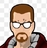### How to use text from textfield in a formulaHello!

I need to calculate a sum of various numbers (ex. sum of electricity bills of a trimester).

It would be convenient to have a field where i just put the amount of every bill in a string like this:

100+130+120,

and another formula field where the sum of these numbers would be calculated.

Lke in Excel, you know... When you put =100+130+120 and it gives you a sum.

I tried a text field (named ´note´) to put the numbers in, and a formula that contains the name of that field, and even like

`number(note)`

but it does not work )).

Is it possilble and how?

Hi Agassi -

It can be done. So let us start with a text field called BillAmounts. Now in this field you can enter in the numbers you want. You need to decide on a consistant delimiter between the numbers. We can use "," for this example, but you can use anything that makes sense to you.

Then you can create a new formula field, call it TotalBillAmounts, and put the following:

let newArray := split(BillAmounts,",") <-- this turns your text field into an array so you can do fun stuff

sum(newArray) <--thiis is self explainitory

If you don't use "," change the first line to whatever you use. You can only put numbers in or the sum function will break.

Good luck and let us know how it go.Hello, Fred!

It works perfectly.

I used a line break instead of a comma.

Its so much easier now with your solution.

Thanjs again!!!Hello again!

Im still thinking if its possible to do calculation of any math string?

I mean - if I put a string 100+40/3 in a formula field, that would be calculated.

But is there a way to put that string in another field,  supposedly a text field, and the formula filed will "look" in that field and calculate the string?

Agassi

If the Text field "MyFormula" contains a formula, the formula "eval(MyFormula, this)" in a Formula field will read and interpret it.Alain, thank you very much!

It works perfect!

So both solutions are good for differrent situations!

Thank you Fred and Alain!!